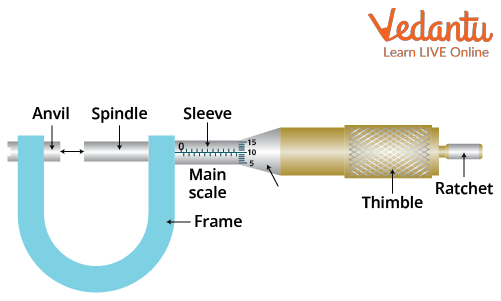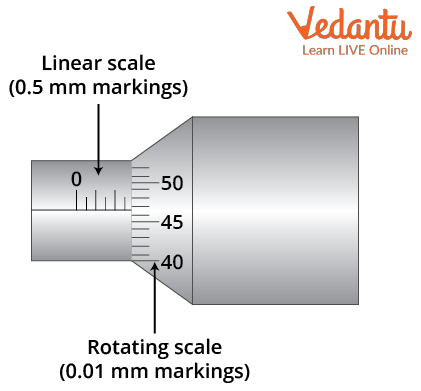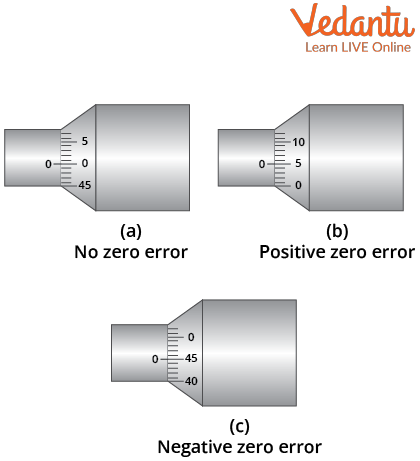Courses
Courses for Kids
Free study material
Free LIVE classes
More

# Micrometre Screw Gauge - Principle and Working for JEELIVE
Join Vedantu’s FREE Mastercalss

## Micrometre Screw Gauge

A micrometre screw gauge is a tool with a calibrated screw that is frequently used for precise measurement of components in mechanical engineering, machining, and most mechanical trades. It is used alongside other meteorological instruments such as dials, verniers, and digital callipers. It is a tool that is commonly used to measure extremely small dimensions.

A micrometre is a neoclassical Greek coinage word, with micros meaning "small" and metron meaning "measure." A micrometre screw gauge is a device calibrated to measure a small length with high accuracy because it has the lowest least count. For example, a screw gauge can be used to measure the diameter of a wire or a thin rod. It can measure up to 10 m or 0.01 mm, which is why it is referred to as a micrometre.

## What Is Micrometre Screw Gauge

A micrometre screw gauge is a tool used to make precise linear measurements of solid body dimensions such as diameter, thickness, and length. It has a C-shaped frame with a movable jaw that is operated by an integral screw. The fineness of the measurement is determined by the lead of the screw, whereas the accuracy of the measurement is determined by the screw-nut combination.

A micrometre screw gauge is usually used to measure the diameter of thin wires or the thickness of small sheets of glass, plastic, or any other sheet that is used in experiments. It is also used in telescopes or microscopes in order to measure the apparent diameter of celestial bodies or microscopic objects through microscopes.

## Principle of Micrometre Screw Gauge

• The screw and thread principle is used by micrometre screw gauges. The screw is attached to a thimble or concentric cylinder with an equal circumference. When a screw is rotated through a nut one revolution, the axial movement is equal to the pitch of the screw's thread.

• If 'I' is the screw-in pitch or lead in mm, then each rotation of the screw advances it by 'I' mm in relation to the internal threads.

• If the circumference of the concentric cylinder is divided into 'n' equal divisions, movement (rotation) of the cylinder through one division represents 1/n revolution of the screw or 1/n mm axial advance.

• Since the screw in most micrometre instruments has a pitch (lead) of 0.5 mm and a thimble has 50 divisions, the micrometre's least count is equal to 0.5/50 = 0.01 mm.

• Lowering the pitch of the screw thread or increasing the number of divisions on the thimble can reduce the axial advance value per one circumferential division and improve measurement accuracy.

## Micrometre Screw Gauge  Least Count

What is least count? The smallest value that a measuring instrument can measure is referred to as its least count. A screw gauge calculates the result using two formulas. The screw gauge's pitch or the screw gauge with the fewest counts is used.

## Micrometre Least Count Formula

Least count of the screw gauge – The ratio of pitch and the total number of divisions can be used to calculate the least count of the screw gauge. The equation is as follows:

$\text{Least count of micrometre}=\dfrac{\text{(Pitch)}}{\text{ total number of divisions}}$

## Digital Micrometre Least Count

Digital micrometres operate differently than mechanical micrometres. A capacitor is used to measure the distance in a digital micrometre. So the least count is determined by the capacitor's ability to measure displacement. The LCD displays provided in digital micrometres display the least count of the device to be used. They are efficient and handy to use because calculations can be skipped. The workpiece is to be held between the anvil and the spindle. The device will show the reading on its own.

## Pitch of Screw Gauge

The screw gauge pitch is the ratio of the distance moved by a screw to the number of rotations. The pitch formula is illustrated below:

$\text{Pitch of the screw gauge}=\frac{\text{(moving distance by a screw)}}{\text{(number of rotations)}}$$\text{Pitch of the screw gauge}=\dfrac{\text{(moving distance by a screw)}}{\text{(number of rotations)}}$.

## Micrometre Screw Gauge Measurements

A screw gauge can be used to measure the thickness of thin sheets, the radius of thin wires, as well as the radius of small structures, as we have already discussed above. It's a U-shaped instrument with a screw on it. For beginners, the fine screw gauge can be difficult to use.

The units are measured using two scales:

1. Pitch scale

2. Circular scale

The primary scale is the pitch scale, which is represented by a vertical line attached to the instrument. The units are measured in millimetres per revolution. A horizontal circular scale is used. A micrometre screw gauge is a circular scale in displacement that is half the length of a millimetre.

## Procedure to Take a Reading

A micrometre screw gauge can be used to measure small (>2.5 cm) diameters that can fit within the screw-'jaws' gauge to within a hundredth of a millimetre. Close the micrometre jaws and look for a zero error. It depicts the various components of a screw gauge.Screw Gauge

As shown in the diagram, place the wire between the anvil and the spindle end. Turn the thimble until the wire is securely held between the anvil and spindle. The ratchet is provided to prevent excessive strain on the wire, which helps to avoid damage to the wire.Screw Gauge diagram for recording a reading

Look at the main scale first. This is a linear scale reading. The long lines represent millimetres, while the shorter ones represent half a millimetre in between. This reading is 2.5 mm on the diagram. Examine the rotating scale now. It represents 46 divisions - each division is 0.01mm, for a total of 0.46mm on this scale. The wire's diameter is the sum of these measurements: 2.5 + 0.46 = 2.96 mm

You can read to half a rotating scale division, or to within 0.005mm, but no further. That assumes you have experience and confidence in using the instrument +/- one division!

## Zero Errors in Micrometre Screw Gauge

There is no error when both ends (stud and spindle) of the screw gauge each other in such a way that the zeros of both the circular scale and the main scale exactly overlap. Otherwise, the instruments have a problem known as zero error. A zero error can be either positive or negative. It displays various types of screw gauge errors.

• Screw gauge negative zero error: The error is negative if the zero of the circular scale is above the index line (reference) line. So, zero correction will be positive.

• Screw gauge positive zero error: If the circular scale's zero is below the reference or index line, the zero error will be positive. So, zero correction will be positive.Diagram for different types of errors

## Conclusion

In short, a micrometre screw gauge is a device widely used for measuring extremely small dimensions. Therefore, we discussed how a screw gauge is used to take a reading. We have learned the working principle of the screw gauge and the calculation of the least count of the screw gauge. Some examples are also explained for better understanding. We have also discussed how we can take a reading using a screw gauge and the sensitivity of the reading. This article is a complete guide to learning the basics of micrometre.

Last updated date: 24th Sep 2023
Total views: 133.5k
Views today: 1.33k

## FAQs on Micrometre Screw Gauge - Principle and Working for JEE

1. Describe the term "precision instruments."

Precision instruments, such as vernier callipers and screw gauges, can measure the smallest fraction of a millimetre. In terms of a micrometre, it is defined as an instrument for precise measurement of small distances, and the sleeve, which will move the spindle by turning, is seen to be graduated with the scale divisions that provide us a measuring accuracy of about (0.01) mm. A vernier calliper is used as an instrument that measures the internal and external dimensions and distances.

2. What is a backlash error?

A backlash error occurs when, after changing the thimble's rotational direction, the screw's tip does not immediately begin to move in the opposite direction but instead remains stationary for a portion of the revolution. The screw threads' deterioration is the cause of this. The screw should only be spun in one direction when collecting measurements in order to prevent a backlash error. If the screw's rotational orientation needs to be altered, it should first be stopped for a while and then rotated counterclockwise.